## 4.1.2 Boundary Conditions

DIRICHLET boundary conditions are introduced at the source, drain, and gate contacts. Potentials are conveniently measured relative to the source potential. The amount of bending of the vacuum enegy level along the length of the CNT is given by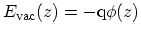, since we assume that the local electrostatic potential rigidly shifts the CNT band-structure. The conduction and valence band-edges of the CNT are given by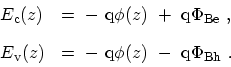(4.6)

The SCHOTTKY barrier heights for electrons (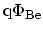) and holes (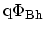) at the metal-CNT interface are given by (see Fig. 4.3)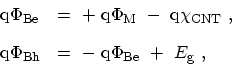(4.7)

where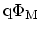is the work function of the metal contact,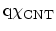is the electron affinity of the CNT, and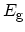is the band-gap of the CNT. The work function of CNT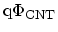is defined as the sum of the CNT electron affinity and half of the band-gap in the bulk. Figure 4.3 shows the band diagram at the metal-CNT interface with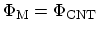. The work function of the CNT is assumed to be. In an intrinsic CNT (un-doped) the FERMI level of the CNT is located in the middle of the band-gap. Under these conditions, equal SCHOTTKY barrier heights for both electrons and holes are achieved. If the work functions of metal and CNT do not align, band-bending near the contact occurs and SCHOTTKY barrier heights for electrons and holes will be different. For example, if the work function of the metal contact is larger than that of the CNT (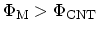) the SCHOTTKY barrier height for holes is smaller than that for electrons. As a result, a p-type CNT-FET is achieved, where holes are the majority carriers.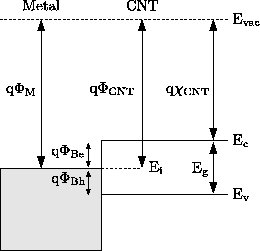M. Pourfath: Numerical Study of Quantum Transport in Carbon Nanotube-Based Transistors